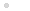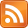### My CPU

Homebrew CPU construction / házi készítésű processzor

### Feedek## FunCPU - Evaluation Strategy

### 2014.07.22. 16:52 Budapesti álmodozó

It is not trivial how expressions should be reduced, i.e. evaluated. I have already mentioned that while some strategy could be fruitful in reducing an expression in a given interpretation, others may be not.

Let us have a look at the following simple example of evaluation of the factorial function is defined under the alias fac.
fac(n):= if n=0 then 1 else n*fac(n-1)

The reduction of fac(1) may lead to

if 1=0 then 0 else 1*fac(0)
If 1=0 then 0 else 1*(if 0=0 then 1 else 0*fac(-1))
If 1=0 then 0 else 1*(if 0=0 then 1 else 0*(if -1=0 then 0 else -1*fac(-1))) .......

We can easily see that the reduction goes on forever, it never terminates.

The goals of the evaluation strategy are as follows:

• Must terminate (simple applicative rules may result in infinite loop)
• Must be efficient (as possible).
• Must be simple (suitable to be represented in hardware directly).

The following strategy will be applied:

A user-defined function is reduced if and only if all of its arguments are constants. Similarly, inc and dec are reduced only, if their arguments are literals. As far as if-then-else is concerned: if its condition part is constant 0 (i.e. true), then it is reduced to exp1. If the condition part is a constant other than 0, then it is reduced to exp2. Othewise the reduction is postponed.

Corollary: all parameters passed to functions are constants.

The reduction algorithm is as follows:

1. Scan input expression symbol by symbol.
2. Copy symbol from source to output if symbol is not a function, or its a function but cannot be reduced in its current form (i.e. contains at least one argument, which is not a constant).
3. Evaluate functions (if, inc, dec, and user-defined) if possible. That is, rewrite the function call by replacing the call with the function definition with bounded arguments.
4. If EOX symbol is found, then the whole cycle restarts. Note: in the next cycle: the current output becomes the input and vice versa.
5. Stop, if the first symbol is a constant. This literal is the result of the reduction.

It is clear that inc, dec terminates immediately, thus reduces the length of expression. The expression size also shrinks when in the expression „if cond exp1 exp2” cond is a constant. Eventually everything is based on/can be reduced to the three built-in functions. Therefore sooner or later, all „if”, „inc”, „dec” are evaluated/reduced, thus the evaluation terminates (if this is possible).

### A bejegyzés trackback címe:

https://mycpu.blog.hu/api/trackback/id/tr76500657

### Kommentek:

A hozzászólások a vonatkozó jogszabályok  értelmében felhasználói tartalomnak minősülnek, értük a szolgáltatás technikai  üzemeltetője semmilyen felelősséget nem vállal, azokat nem ellenőrzi. Kifogás esetén forduljon a blog szerkesztőjéhez. Részletek a  Felhasználási feltételekben és az adatvédelmi tájékoztatóban.

Nincsenek hozzászólások.